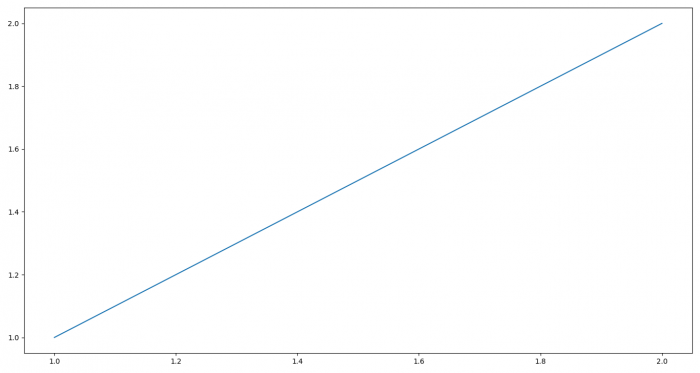# Show Matplotlib graphs to image as fullscreen

To show matplotlib graphs as full screen, we can use full_screen_toggle() method.

## Steps

• Create a figure or activate an existing figure using figure() method.

• Plot a line using two lists.

• Return the figure manager of the current figure.

• To toggle full screen image, use full_screen_toggle() method.

• To display the figure, use show() method.

## Example

from matplotlib import pyplot as plt
plt.rcParams["figure.figsize"] = [7.00, 3.50]
plt.rcParams["figure.autolayout"] = True
plt.figure()
plt.plot([1, 2], [1, 2])
manager = plt.get_current_fig_manager()
manager.full_screen_toggle()
plt.show()

## Output